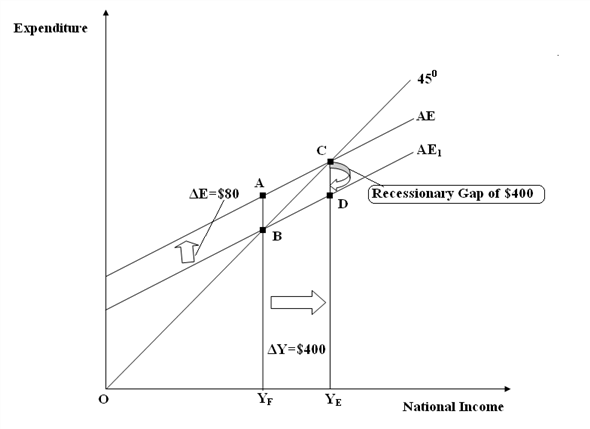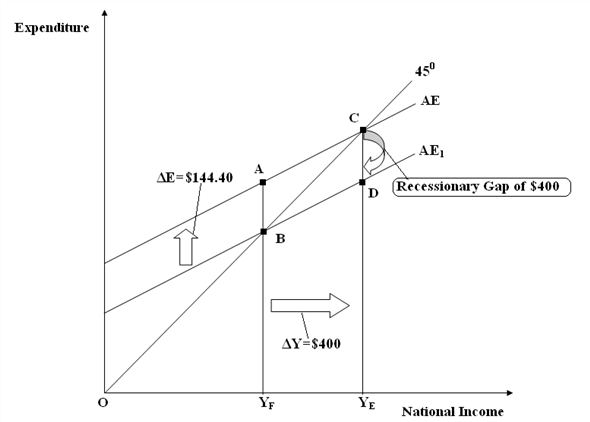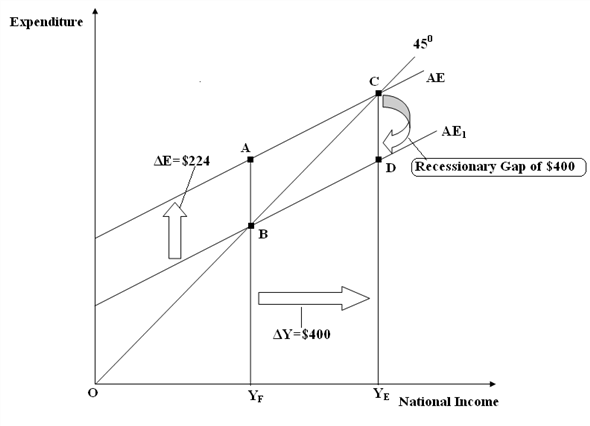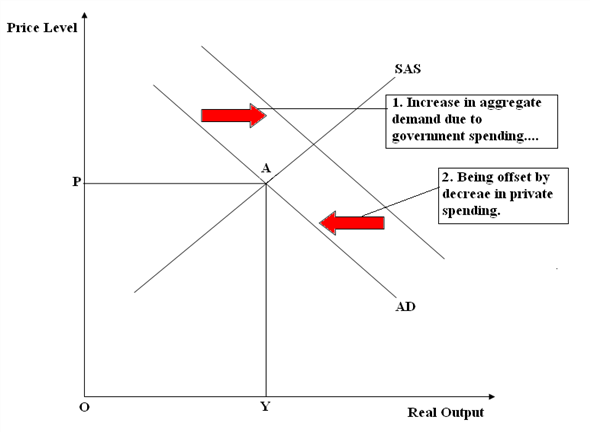# Quiz 18: International Trade Policy

(a) It is given that, • Value of mpe is 0.8. • Recessionary gap in the economy is of \$400. It has been stated that, government wants to eliminate this recessionary gap by changing expenditure i.e. by increasing expenditure.First, calculate the value of multiplier.Therefore, the value of multiplier is 5. Elimination of recessionary gap of \$400 implies increase in income by \$400. Therefore, change in income is \$400. With value of multiplier being 5, we have to calculate the change in expenditure required to eliminate this recessionary gap. Calculation of change in expenditure:Therefore, government has to change the expenditure or have to increase the autonomous expenditure by \$80, if it wants to eliminate the recessionary gap of \$400. (b) It has been stated that, government wants to eliminate this recessionary gap by decreasing taxes instead of changing expenditure.First, calculate the value of tax multiplier.Elimination of recessionary gap of \$400 implies increase in income by \$400. Therefore, change in income is \$400. With value of Tax multiplier being 4, calculate the change in taxes required to eliminate this recessionary gap. Calculation of change in taxes:Therefore, government has to change the taxes or have to decrease the taxes by \$100, if it wants to eliminate the recessionary gap of \$400. (c) Given that, mpe = 0.8 and marginal tax rate = 0.2, then multiplier is:Elimination of recessionary gap of \$400 implies increase in income by \$400. Therefore, change in income is \$400. With value of multiplier being 2.77, calculate the change in expenditure required to eliminate this recessionary gap. Calculation of change in expenditure:Therefore, government has to change the expenditure or have to increase the autonomous expenditure by \$144.40 , if it wants to eliminate the recessionary gap of \$400. It has been stated that, government wants to eliminate this recessionary gap by decreasing taxes instead of changing expenditure.First, calculate the value of tax multiplier.Elimination of recessionary gap of \$400 implies increase in income by \$400. Therefore, change in income is \$400. With value of tax multiplier being 2.22, calculate the change in taxes required to eliminate this recessionary gap. Calculation of change in taxes:Therefore, government has to change the taxes or have to decrease the taxes by \$180.18, if it wants to eliminate the recessionary gap of \$400. Therefore, to eliminate recessionary gap of \$400: • Expenditure has to be increased by \$144.40 Or, • Taxes has to be decreased by \$180.18 (d) Given that, • mpe = 0.8 • Marginal tax rate = 0.2 • Marginal propensity to consume = 0.2 The value of multiplier is:Elimination of recessionary gap of \$400 implies increase in income by \$400. Therefore, change in income is \$400. With value of multiplier being 1.79, calculate the change in expenditure required to eliminate this recessionary gap. Calculation of change in expenditure:Therefore, government has to change the expenditure or have to increase the autonomous expenditure by \$224, if it wants to eliminate the recessionary gap of \$400. It has been stated that, government wants to eliminate this recessionary gap by decreasing taxes instead of changing expenditure.First, calculate the value of tax multiplier.Elimination of recessionary gap of \$400 implies increase in income by \$400. So, change in income is \$400. With value of Tax multiplier being 1.43, we have to calculate the change in taxes required to eliminate this recessionary gap. Calculation of change in taxes:Therefore, government has to change the taxes or have to decrease the taxes by \$280 , if it wants to eliminate the recessionary gap of \$400. Therefore, to eliminate recessionary gap of \$400: • Expenditure has to be increased by \$224 Or, • Taxes have to be decreased by \$280. (e) Following figure shows the elimination of recessionary gap of \$400, when expenditure is increased by \$80 (part a):Initially, economy is operating at the full-employment or potential level of output Y E with aggregate demand curve AE intersecting 45 0 line at point c.Now, due to some adverse happening in the economy of Textland (say due to crash in the stock market), there is decline in investment. With decline in investment, say equal to CD, aggregate demand curve will shift downward to the new position AE 1, which will bring the economy to new equilibrium point at B and thereby determine the Y F level of income. This fall in income will led to the emergence of recessionary gap of \$400 in the economy of Textland.It has been stated that, government wants to eliminate this recessionary gap by increasing expenditure. With mpe of 0.8 and multiplier of 5, economy requires an increase in autonomous expenditure of \$80 or decrease in taxes of \$100 to eliminate this recessionary gap of \$400. In the above figure, it has been observed that, when expenditure increases by \$80, the aggregate demand curve shifts upward to its original position i.e. from AE 1 to AE. As a result, the equilibrium level of income will increase to full-employment or potential level of output Y E and in this way, recessionary gap has been eliminated.Following figure shows the elimination of recessionary gap of \$400, when expenditure is increased by \$144.40 (part c):Initially, economy is operating at the full-employment or potential level of output Y E with aggregate demand curve AE intersecting 45 0 line at point c.Now, due to some adverse happening in the economy of Textland (say due to crash in the stock market), there is decline in investment. With decline in investment, say equal to CD, aggregate demand curve will shift downward to the new position AE 1 , which will bring the economy to new equilibrium point Band thereby determine the Y F level of income. This fall in income will led to the emergence of recessionary gap of \$400 in the economy of Textland.It has been stated that, government wants to eliminate this recessionary gap by increasing expenditure. With mpe of 0.8 and multiplier of 2.77, economy requires an increase in autonomous expenditure of \$144.40 or decrease in taxes of \$180.18 to eliminate this recessionary gap of \$400. In the above figure, it has been observed that, when expenditure increases by \$144.40, the aggregate demand curve shifts upward to its original position that is from AE 1 to AE. As a result, the equilibrium level of income will increase to full-employment or potential level of output Y E and in this way, recessionary gap has been eliminated.Following figure shows the elimination of recessionary gap of \$400, when expenditure is increased by \$224 (part d):Initially, economy is operating at the full-employment or potential level of output Y E with aggregate demand curve AE intersecting 45 0 line at point c.Now, due to some adverse happening in the economy of Textland (say due to crash in the stock market), there is decline in investment. With decline in investment, say equal to CD, aggregate demand curve will shift downward to the new position AE 1 , which will bring the economy to new equilibrium point Band thereby determine the Y F level of income. This fall in income will led to the emergence of recessionary gap of \$400 in the economy of Textland.It has been stated that, government wants to eliminate this recessionary gap by increasing expenditure. With mpe of 0.8 and multiplier of 1.79, economy requires an increase in autonomous expenditure of \$224 or decrease in taxes of \$280 to eliminate this recessionary gap of \$400. In the above figure, it has been observed that, when expenditure increases by \$224, the aggregate demand curve shifts upward to its original position i.e. from AE 1 to AE. As a result, the equilibrium level of income will increase to full-employment or potential level of output Y E and in this way, recessionary gap has been eliminated.
(a) According to the Ricardian equivalence theorem, there would be no effect of deficit spending on the interest rate and private investment. This implies that even if government finance it is spending by selling bonds there would be no increase in interest rates and thus there would be no crowding out of private investment. Following figure shows the market forAs above figure shows, initiallyNow, government finance it is spending by selling bonds, this selling of bonds implies rise in demand forAs Ricardian equivalence theorem states that if government finance current spending by running deficit that is by selling bonds then, in future, it have to raise taxes so that it can have necessary resources to pay for the interest on the bonds and to repay the bonds when they came to maturity. Furthermore, to pay for the increased taxes in future, people will now consume less and would save more. These savings in anticipation of future taxes will meet the rise in demand forNew equilibrium is attained at point B, where interest rate is R percent per year and quantity ofThus, interest rate has remained same.Since, interest rate has remained same, no crowding out effect has happened and therefore private investment will remain same.(b) According to Ricardian equivalence theorem, there would be no effect of deficit spending on the output in the economy. This happens because all income accruing to the households and firms from the debt financed government expenditure will be saved in anticipation of future tax burden and thus does not lead to increase in demand for goods and services by households and firms and therefore output in the economy remains same and there is no expansion of aggregate demand.Following figure shows the Goods market -As above figure shows, initially output in the economy is Y. Now, government increases its spending by running deficit that is by selling bonds. This selling of bonds by government to meet its current spending implies that in future taxes will be raised so that necessary resources could be generated to pay the interest on bonds as well as bonds upon maturity. This anticipation of increase in taxes in future will compel the people to decrease their current consumption and increase their current savings so that they would be able to pay the high taxes in future. Thus, increase in government spending will exactly be offset by the equivalent decrease in private spending and hence aggregate demand or aggregate expenditure will remain same and therefore output in the economy remains same.This shown in the above figure by the rightward shift of the aggregate demand curve from AD to AD 1 due to increase in government spending and then back to AD itself due to equivalent decline in private spending. So, output in the economy has remained same.